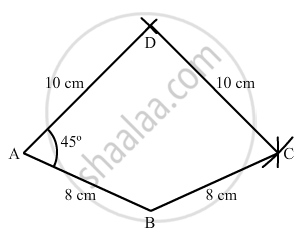Share

# Construct a Quadrilateral Abcd, Given that Ab = 8 Cm, Bc = 8 Cm, Cd = 10 Cm, Ad = 10 Cm and ∠A = 45°. - Mathematics

Course
ConceptConstructing a Quadrilateral When Two Adjacent Sides and Three Angles Are Known

#### Question

Construct a quadrilateral ABCD, given that AB = 8 cm, BC = 8 cm, CD = 10 cm, AD = 10 cm and ∠A = 45°.

#### Solution

Steps of Construction:

Step I: Draw AB = 8 cm .

$\text { Step II: Construct } \angle BAD = 45^\circ.$

Step III : With A as the centre and radius 10 cm, cut off AD = 10 cm .

Step IV: With D as the centre and radius 10 cm, draw an arc .

Step V : With B as the centre and radius 8 cm, draw an arc to intersect the arc drawn in Step IV at C .

Step VI: Join BC and CD to obtained the required quadrilateral .Is there an error in this question or solution?

#### APPEARS IN

RD Sharma Solution for Mathematics for Class 8 by R D Sharma (2019-2020 Session) (2017 to Current)
Chapter 18: Practical Geometry (Constructions)
Ex. 18.3 | Q: 2 | Page no. 8
Solution Construct a Quadrilateral Abcd, Given that Ab = 8 Cm, Bc = 8 Cm, Cd = 10 Cm, Ad = 10 Cm and ∠A = 45°. Concept: Constructing a Quadrilateral - When Two Adjacent Sides and Three Angles Are Known.
S